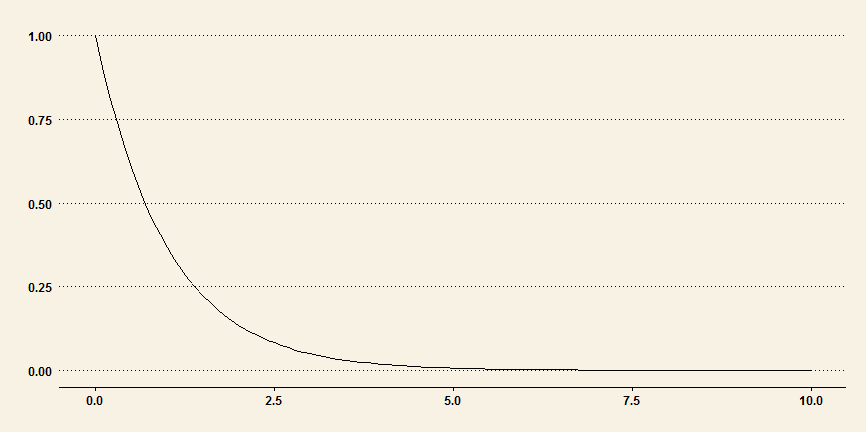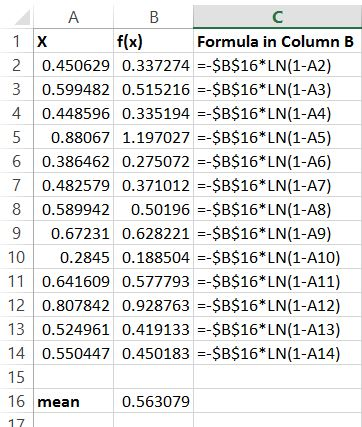# Excel Exponential Distribution

Generating random numbers from the exponential distribution in Excel should not be such a difficult task, but the lack of a direct function does it make it difficult. In this post, you will see the steps to generate random numbers from the exponential distribution in Excel.## Understanding Exponential Distribution

The probably density function (PDF) of exponential distribution is:
`f(x; lambda) = lambda * exp(-lambda * x)`

where:

x is greater than or equal to zero

lambda is the rate parameter or 1/mean.

The cumulative distribution function (CDF) of exponential distribution is:

`F(x; lambda) = 1 - exp(-lambda * x)`

Percent Point Function (PPF) or Inverse of the cumulative distribution function, given by:

`G(x) = - 1/lambda * logn(1- x)`

We will use the PPF to generate exponential distribution random numbers.

## Step 1: Generate Random Numbers from Uniform Distribution

• The first step is to create a set of uniform random numbers between 0 and 1. To generate these random numbers, simple enter this following command in your Excel sheet cell A2:

`=RAND()`

• Copy the formula down to A21, so that we have 20 random numbers from A2:A21.

Step 2: Calculate Mean of the Random Numbers

In a blank cell, say A22, calculate the mean of the numbers. Enter this formula:

`=AVERAGE(A2:A21)`

## Step 2: Generate Random Numbers from Exponential Distribution

• In cell B2, enter this formula (there is a minus sign in front of A22):

`=-A22 * LN(1 - A2)`

where:

LN is the natural logarithm value.

• In the formula, make A22 absolute value by place the \$ sign in front of the letter and numbers, such as \$A\$22.
• Drag the formula down to B21

See this following image for an example:There you have it: you have successfully generated random numbers in Excel from the exponential distribution.

### Excel And Statistics Books You May Be Interested In:

Sale
Applied Statistics for Business and Management using Microsoft Excel
• Herkenhoff, Linda (Author)
• English (Publication Language)
• 431 Pages - 11/26/2013 (Publication Date) - Springer (Publisher)
Sale
Microsoft Excel Data Analysis and Business Modeling
• Microsoft Press
• Winston, Wayne (Author)
• English (Publication Language)
• 864 Pages - 12/09/2016 (Publication Date) - Microsoft Press (Publisher)
Sale
• Winston, Wayne (Author)
• English (Publication Language)
• 880 Pages - 04/15/2019 (Publication Date) - Microsoft Press (Publisher)
Excel for Beginners: Learn Excel 2016, Including an Introduction to Formulas, Functions,...
• Shields, Greg (Author)
• English (Publication Language)
• 80 Pages - 09/06/2018 (Publication Date) - CreateSpace Independent Publishing Platform (Publisher)

Last update on 2020-11-24 / Affiliate links / Images from Amazon Product Advertising API

•Gordon says:
•Dale says: